Related Articles

# Easy way to remember Strassen’s Matrix Equation

• Difficulty Level : Medium
• Last Updated : 14 Jul, 2020

Strassen’s matrix is a Divide and Conquer method that helps us to multiply two matrices(of size n X n).

You can refer to the link, for having the knowledge about Strassen’s Matrix first :
Divide and Conquer | Set 5 (Strassen’s Matrix Multiplication)

But this method needs to cram few equations, so I’ll tell you the simplest way to remember those :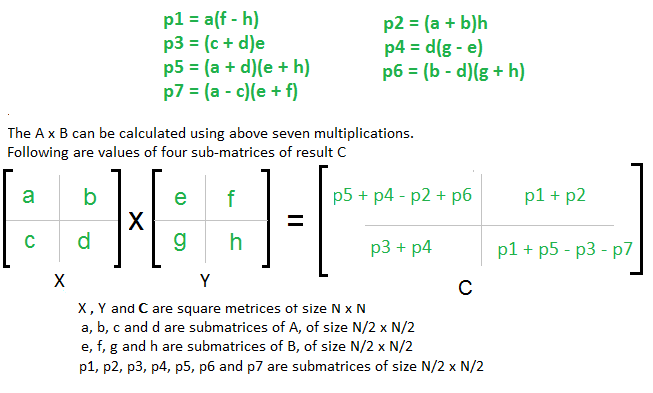You just need to remember 4 Rules :

• AHED (Learn it as ‘Ahead’)
• Diagonal
• Last CR
• First CR

Also, consider X as (Row +) and Y as (Column -) matrix

• Write P1 = A; P2 = H; P3 = E; P4 = D
• For P5 we will use Diagonal Rule i.e.
(Sum the Diagonal Elements Of Matrix X ) * (Sum the Diagonal Elements Of Matrix Y ), we get
P5 = (A + D)* (E + H)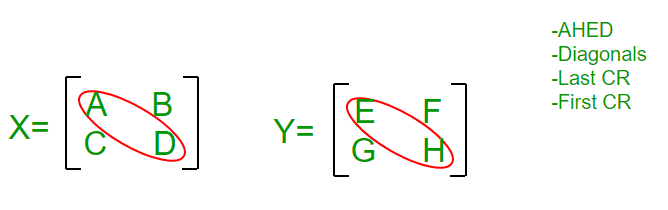P1 = A
P2= H
P3= E
P4= D
P5= ( A + D ) * ( E + H )

• For P6 we will use Last CR Rule i.e. Last Column of X and Last Row of Y and remember that Row+ and Column- so i.e. (B – D) * (G + H), we get
P6 = (B – D) * (G + H)
• For P7 we will use First CR Rule i.e. First Column of X and First Row of Y and remember that Row+ and Column- so i.e. (A – C) * (E + F), we get
P7 = (A – C) * (E + F)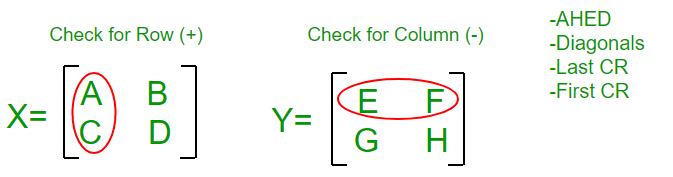P1 = A
P2= H
P3= E
P4= D
P5= ( A + D ) * ( E + H )
P6= ( B – D ) * ( G + H)
P7= ( A – C ) * ( E + F)

• Come Back to P1 : we have A there and it’s adjacent element in Y Matrix is E, since Y is Column Matrix so we select a column in Y such that E won’t come, we find F H Column, so multiply A with (F – H)
So, finally P1 = A * (F – H)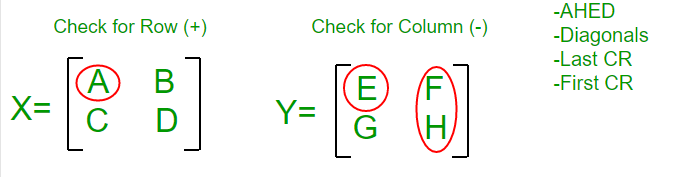P1 = A * ( F – H)
P2= H
P3= E
P4= D
P5= ( A + D ) * ( E + H )
P6= ( B – D ) * ( G + H)
P7= ( A – C ) * ( E + F)

• Come Back to P2 : we have H there and it’s adjacent element in X Matrix is D, since X is Row Matrix so we select a Row in X such that D won’t come, we find A B Column, so multiply H with (A + B)
So, finally P2 = (A + B) * H
• Come Back to P3 : we have E there and it’s adjacent element in X Matrix is A, since X is Row Matrix so we select a Row in X such that A won’t come, we find C D Column, so multiply E with (C + D)
So, finally P3 = (C + D) * E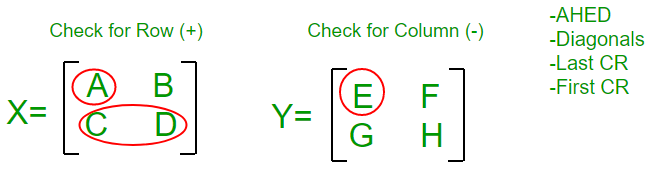P1= A * ( F – H )
P2= H * ( A + B )
P3= E * ( C + D )
P4= D
P5= ( A + D ) * ( E + H )
P6= ( B – D ) * ( G + H)
P7= ( A – C ) * ( E + F)

• Come Back to P4 : we have D there and it’s adjacent element in Y Matrix is H, since Y is Column Matrix so we select a column in Y such that H won’t come, we find G E Column, so multiply D with (G – E)
So, finally P4 = D * (G – E)

We are done with P1 – P7 equations, so now we move to C1 – C4 equations in Final Matrix C :

• Remember Counting : Write P1 + P2 at C2
• Write P3 + P4 at its diagonal Position i.e. at C3
• Write P4 + P5 + P6 at 1st position and subtract P2 i.e. C1 = P4 + P5 + P6 – P2
• Write odd values at last Position with alternating – and + sign i.e. P1 P3 P5 P7 becomes
C4 = P1 – P3 + P5 – P7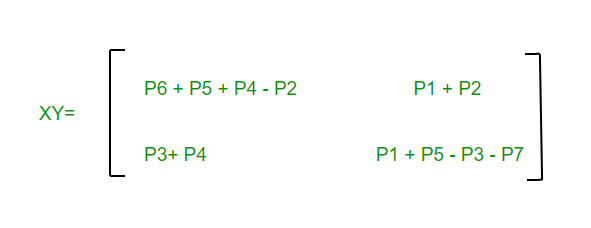This article is contributed by Mohit Gupta 🙂. If you like GeeksforGeeks and would like to contribute, you can also write an article using contribute.geeksforgeeks.org or mail your article to contribute@geeksforgeeks.org. See your article appearing on the GeeksforGeeks main page and help other Geeks.